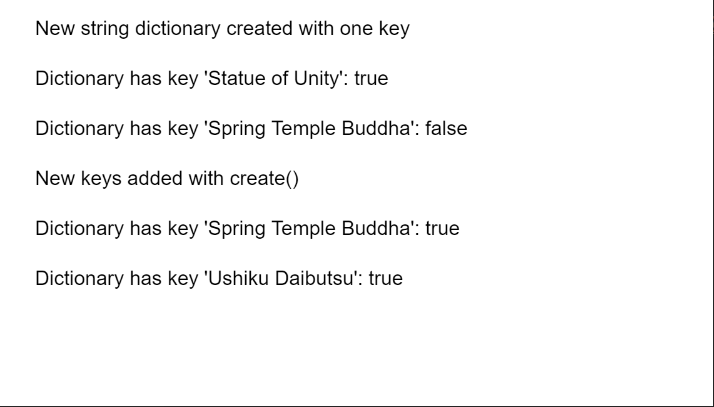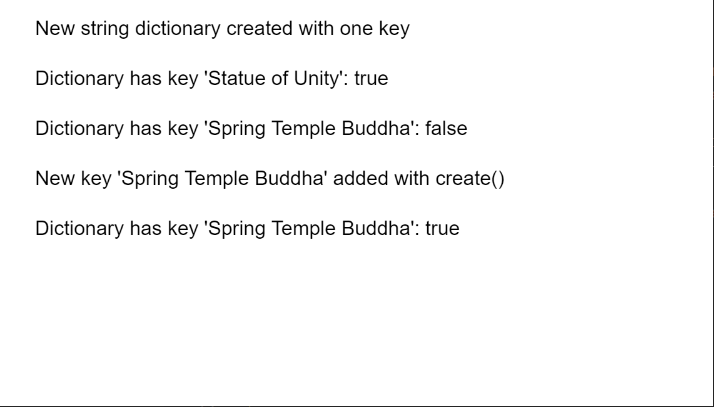Open in App
Not now

# p5.js TypedDict create() Method

• Last Updated : 25 Nov, 2020

The create() method of p5.TypedDict in p5.js is used to add the given key-value pair or collection of pairs to the dictionary. A key-value pair is a set of two values that are mapped to each other. These values can be accessed by querying this dictionary using the key portion of the pair. A dictionary can store multiple key-value pairs that can be accessed using the methods of the dictionary.

Syntax:

```create( key, value )
```

or

```create( obj )
```

Parameters:

• key: It specifies the string that is used as the key to be added to the dictionary.
• value:It specifies the string that is used as the value to be added to the dictionary.
• obj: It specifies the object that contains the key-value pairs to be added to the dictionary.

The example below illustrates the create() method in p5.js:

Example 1:

## Javascript

 `function` `setup() { ` `  ``createCanvas(550, 300); ` `  ``textSize(16); ` ` `  `  ``let stringDict = ` `      ``createStringDict(``"Statue of Unity"``, ``"182 m"``); ` `  ``text(``"New string dictionary created "` `+ ` `       ``"with one key"``, 20, 20); ` ` `  `  ``let existOne =  ` `      ``stringDict.hasKey(``"Statue of Unity"``); ` `  ``text(``"Dictionary has key "` `+ ` `       ``"'Statue of Unity': "` `+ ` `       ``existOne, 20, 60); ` ` `  `  ``let existTwo =  ` `      ``stringDict.hasKey(``"Spring Temple Buddha"``); ` `  ``text(``"Dictionary has key "` `+ ` `       ``"'Spring Temple Buddha': "` `+ ` `       ``existTwo, 20, 100); ` ` `  `  ``let tmpObj = { ` `    ``"Spring Temple Buddha"``: ``"128 m"``, ` `    ``"Ushiku Daibutsu"``: ``"100 m"``, ` `    ``"Great Buddha of Thailand"``: ``"92m"` `  ``}; ` ` `  `  ``// Add the given key to the dictionary ` `  ``// specifying the key and value as an object ` `  ``stringDict.create(tmpObj); ` `  ``text(``"New keys added with create()"``, ` `       ``20, 140); ` ` `  `  ``existTwo =  ` `    ``stringDict.hasKey(``"Spring Temple Buddha"``); ` `  ``text(``"Dictionary has key "` `+ ` `       ``"'Spring Temple Buddha': "` `+ ` `       ``existTwo, 20, 180); ` ` `  `  ``let existThree = ` `      ``stringDict.hasKey(``"Ushiku Daibutsu"``); ` `  ``text(``"Dictionary has key "` `+ ` `       ``"'Ushiku Daibutsu': "` `+  ` `       ``existThree, 20, 220); ` `}`

Output:Example 2:

## Javascript

 `function` `setup() { ` `  ``createCanvas(550, 300); ` `  ``textSize(16); ` ` `  `  ``let stringDict = ` `      ``createStringDict(``'Statue of Unity'``, ` `                       ``'182 m'``); ` `  ``text(``"New string dictionary "` `+ ` `       ``"created with one key"``, 20, 20); ` ` `  `  ``let existOne = ` `      ``stringDict.hasKey(``'Statue of Unity'``); ` `  ``text(``"Dictionary has key 'Statue of Unity': "` `        ``+ existOne, 20, 60); ` ` `  `  ``let existTwo = ` `      ``stringDict.hasKey(``'Spring Temple Buddha'``); ` `  ``text(``"Dictionary has key "` `+ ` `       ``"'Spring Temple Buddha': "` `+ ` `       ``existTwo, 20, 100); ` ` `  `  ``// Add the given key to the dictionary ` `  ``// specifying the key and value ` `  ``stringDict.create(``'Spring Temple Buddha'``, ` `                    ``'128 m'``); ` `  ``text(``"New key 'Spring Temple Buddha'"` `+ ` `       ``" added with create()"``, 20, 140) ` ` `  `  ``existTwo =  ` `    ``stringDict.hasKey(``'Spring Temple Buddha'``); ` `  ``text(``"Dictionary has key "` `+ ` `       ``"'Spring Temple Buddha': "` `+ ` `       ``existTwo, 20, 180); ` `}`

Output:Online editor: https://editor.p5js.org/
Environment Setup: https://www.geeksforgeeks.org/p5-js-soundfile-object-installation-and-methods/
Reference: https://p5js.org/reference/#/p5.TypedDict/create

My Personal Notes arrow_drop_up
Related Articles